# RD Sharma Solutions for Class 6 Maths Chapter 17 Symmetry Exercise 17.4

## RD Sharma Solutions Class 6 Chapter 17 Exercise 17.4

#### Exercise 17.4

1. The total number of lines of symmetry of a scalene triangle is

Soln: (d) none of these

This is because the line of symmetry of a scalene triangle is 0.

2. The total number of lines of symmetry of an isosceles triangle is

Soln: (a) 13. An equilateral triangle is symmetrical about each of its

Soln: (d) all the above

In equilateral triangle altitudes, angle bisectors and medians are all the same.4. The total number of lines of symmetry of a square is

Soln: (d) 4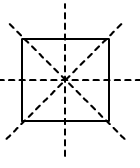5. A rhombus is symmetrical about

Soln: (a) Each of its diagonals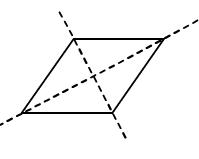6. The number of lines of symmetry of a rectangle is

Soln: (b) 2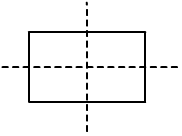7. The number of lines of symmetry of a kite is

Soln: (b) 18. The number of lines of symmetry of a circle is

Soln: (d) Unlimited

A circle has an infinite number of symmetry all along the diameters. It has an infinite number of diameters9. The number of lines of symmetry of a regular hexagon is

Soln: (c) 6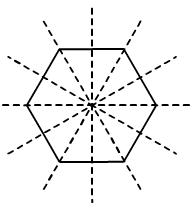10. The number of lines of symmetry of an n – sided regular polygon is

Soln: (a) n

The number of lines of symmetry of a regular polygon is equal to the sides of the polygon. If it has ‘n’ number of sides, then there are ‘n’ lines of symmetry

11. The number of lines of symmetry of the letter O of the English alphabet is

Soln: (c) 2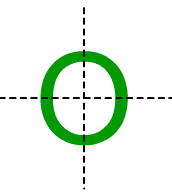12. The number of lines of symmetry of the letter Z of the English alphabet is

Soln: (a) 0

Z has no line of symmetry